Equable Cone

EQUABLE CONE

Balmoral Software

Solutions: 10

For a right circular cone with base radius r and height h, we have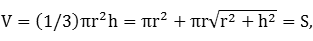which reduces to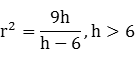Equivalently,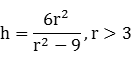The base area must be an integer multiple of π, so let r2 = k for some positive integer k. We then have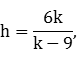so h is rational. The volume V must also be an integer multiple of π, and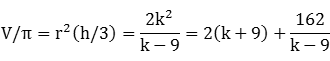Therefore, k - 9 must divide 162, and so r2 ∈ {10,11,12,15,18,27,36,63,90,171}. The following are all 10 equable right cones, ordered by volume:
hr2rLateral surface area/πV/π=S/π
1218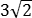4.2435472
1515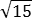3.8736075
927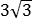5.1965481
83666.0006096
2412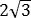3.4648496
3311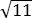3.317110121
763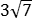7.93784147
20/390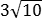9.487110200
6010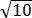3.162190200
19/3171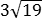13.077190361
The only equable solution where r is also rational is the all-integer solution h = 8 and r = 6, for which V = S = 96 π. That cone is a solid of revolution of an equable right triangle with base 6 and height 8, having perimeter and area equal to 24.